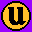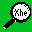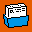# Example of using the shift command

To successively shift the argument that is represented by each positional parameter:

```   cat shift_demo
#!/bin/sh
echo "arg1=\$1 arg2=\$2 arg3=\$3"
shift
echo "arg1=\$1 arg2=\$2 arg3=\$3"
shift
echo "arg1=\$1 arg2=\$2 arg3=\$3"
shift
echo "arg1=\$1 arg2=\$2 arg3=\$3"
shift_demo one two three four five six seven
arg1=one arg2=two arg3=three
arg1=two arg2=three arg3=four
arg1=three arg2=four arg3=five
arg1=four arg2=five arg3=six
arg1=five arg2=six arg3=seven
```